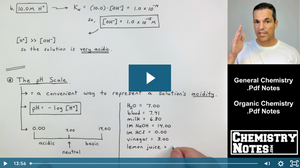# Chemistry Crash Course | Chemistry Help

## S16E3 - Spontaneous Reactions and the Gibbs Free Energy Equation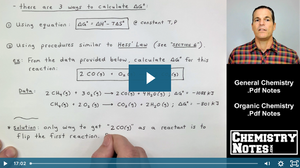Spontaneity and Spontaneous Reactions, the Gibbs Free Energy Equation, and Thermodynamic Calculations. Entropy Calculations and Free Energy Calculations. ΔG.

## S5E3 - Gas Law Examples and Practice Problems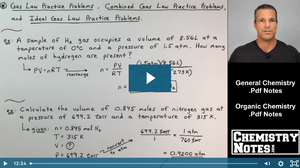Examples of Gas Law Practice Problems, Including Ideal Gas Law Examples, Charles Law Examples, and Combined Gas Law Examples. Practice Problems and Complete Solutions to Various Gas Law Practice Problems.

## S15E3 - The Henderson-Hasselbalch Equation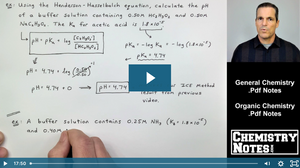The Henderson-Hasselbalch Equation and Titration Curves. How to Solve Acid-Base Titration Examples with the Henderson-Hasselbalch Equation. Titrant vs. Analyte.

## S4E3 - Molarity Calculations. How to Find Molarity of the Solution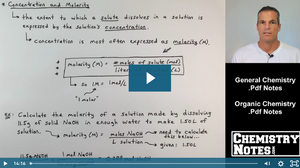Molarity Calculations. How to Find Molarity and Use the Molarity Formula to Solve for Concentration. Also, We'll Do Dilution Problems and the Dilution Formula.